# GED Math : Other Data Calculations

## Example Questions

### Example Question #11 : Other Data Calculations

In 2000 Richard bought a condo for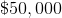. Ten years later he sold it for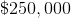. How much did the condo appreciate (grow in value) per year on average (in dollars)?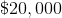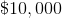Explanation:

To find the amount of growth the condo had per year we first need to find the amount of growht it had total. To find this we subtract the amount he paid from the amount he sold.

The condo grew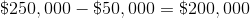in value.

Over 10 years that is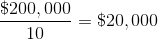per year.

### Example Question #12 : Other Data Calculations

Cynthia madepotato pancakes for Jeff. He loved them so much he ate them all in two days! How many potato pancakes did Jeff eat per day on average?Explanation:

To find out how many pancakes Jeff ate on average we take the total number of pancakes he ate and divide it by the number of days he ate them.

The following fraction is our solution.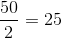### Example Question #13 : Other Data Calculations

Determine the range of the numbers: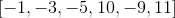Explanation:

The range is the difference of the largest and smallest numbers.

The largest number is 11.

The smallest number is negative nine.

Subtract both quantities.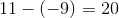The answer is:### Example Question #14 : Other Data Calculations

Use the following data set of test scores to answer the question: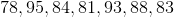Find the range.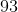Explanation:

To find the range of a data set, we will find the smallest and largest number within the set. Then, we will find the difference of those two numbers.

So, given the setwe can see the smallest number is 78 and the largest number is 95. Now, we will find the difference.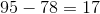Therefore, the range of the data set of test scores is 17.

### Example Question #15 : Other Data Calculations

A Science class took an exam. Here are the scores of 9 students: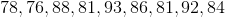Find the range.Explanation:

To find the range of a data set, we will find the smallest and the largest number. Then, we will find the difference of those two numbers.

So, given the setwe can see the smallest number is 76 and the largest number is 93. Now, we will find the difference. We get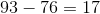Therefore, the range of the data set is 17.

### Example Question #16 : Other Data Calculations

A class took a Math exam.  Here are the test scores of 10 students.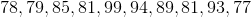Find the range.Explanation:

To find the range of a data set, we will find the smallest and largest number within the set, then we will find the difference of those two numbers.

So, given the data setwe can see the smallest number is 77 and the largest is 99. Now, we will find the difference. We get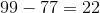Therefore, the range of the data set is 22.

### Example Question #17 : Other Data Calculations

Calculate the range of the following numbers: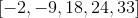Explanation:

The range is the difference between the highest and lowest numbers.

The highest value is 33.

The lowest value is negative nine.

Subtract both quantities.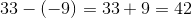The answer is:### Example Question #18 : Other Data Calculations

Identify the range of the following numbers: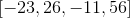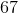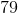Explanation:

The range is the difference between the largest and smallest numbers.

The largest number is:The smallest number is: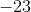Subtract both quantities.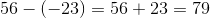The answer is:### Example Question #19 : Other Data Calculations

Determine the range of the numbers: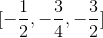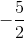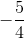Explanation:

The range is the difference between the largest and smallest numbers.

The largest number is:The smallest number is: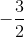Subtract the quantities.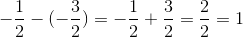The answer is:### Example Question #20 : Other Data Calculations

A class took a Math exam. Here are the test scores of 8 students.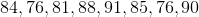Find the range.Explanation:

To find the range of a data set, we will find the smallest and largest number within the set, then we will find the difference of those two numbers.

So, given the data setwe can see the smallest number is 76 and the largest is 91. Now, we will find the difference. We get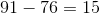Therefore, the range of the data set is 15.

### All GED Math Resources